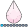## Classify records based on Two columns in SAS Data Step

I need to classify each unique ID based on two columns. One is academic level, and 2nd one is Intensity_FLG. So within each Academic_Level, if the sum of INTENSITY_FLG >0, then this ID should be classified as Full-time, otherwise, this ID will be classified as Part-time. Not sure what goes wrong, the output only populates partial values for that created column ENR_Intensity, and some IDs are still classified based on each row. The Intensity_FLG is based on each record for each ID. For example, U24654 has three records on Intensity_FLG, one of them is 1, so this ID should be classified as Full-time for all three rows. How should I change my code? Thanks.

``````DATA ENR_2;
SET ENR;
``````

4 REPLIES 4

## Re: Classify records based on Two columns in SAS Data Step

Sort your data so that intensity flag is 1 in the first record and retain the flag. SAS does operate on each row individually, so otherwise you need to either calculate the value separately and merge it in or you need to use SQL.

``````proc sort data=have;
run;

data want;
set have;
retain enr_intensity;
if first.academic_level and intensity_flag=1 then enr_intensity='Full-Time';
else enr_intensity='Part-Time';
run;``````

Or add the sum as a new column and use that to classify.

``````proc sql;
create table want as
select *, sum(intensity_flg) as total_intensity
/*,case when total_intensity >=1 then 'Full-time'
else 'Part-Time' end as ENR_INTENSITY*/
from have
group by
uid, intensity_flg;
quit;``````

@xliu1 wrote:

I need to classify each unique ID based on two columns. One is academic level, and 2nd one is Intensity_FLG. So within each Academic_Level, if the sum of INTENSITY_FLG >0, then this ID should be classified as Full-time, otherwise, this ID will be classified as Part-time. Not sure what goes wrong, the output only populates partial values for that created column ENR_Intensity, and some IDs are still classified based on each row. The Intensity_FLG is based on each record for each ID. For example, U24654 has three records on Intensity_FLG, one of them is 1, so this ID should be classified as Full-time for all three rows. How should I change my code? Thanks.

``````DATA ENR_2;
SET ENR;
``````

## Re: Classify records based on Two columns in SAS Data Step

First, lets post something actually readable:

```IF Academic_Level='NonDegree' AND SUM (INTENSITY_FLG)>0 THEN ENR_Intensity='Full-Time';
ELSE IF Academic_Level='NonDegree' AND SUM (INTENSITY_FLG)=0 THEN ENR_Intensity='Part-Time';

If you expect the SUM to be adding values from multiple observations then you are misunderstanding how a DATASTEP SUM (or Mean, STD, Max, Min) function works. You are only referencing one variable, so only the value of that single variable on that single observation is used so "SUM (INTENSITY_FLG)" is exactly equal to INTENSITY_FLG.

Your other main problem likely comes in from this:

```IF SUM(LEVEL_FLG) <0 THEN Academic_Level='NonDegree';

If the variable Academic_level does not exist on the input data set then it is created on the first line of that IF. Since you do not specify a length for the variable before creation SAS uses the length of the first value "NonDegree" to set the length.

Which is shorter than Undergraduate. So the value is truncated to "Undergrad", matching the length of NonDegree.

When attempt to compare the value of the Academic_level = "Undergraduate" that fails because that is not the value of the variable and is never true.

add this line BEFORE the If to specify a length.

`Length Academic_Level \$ 13;`

Example input data in the form of a data step and what you expect for output given that data step might give us a chance to provide what you need.HB
Barite | Level 11

## Re: Classify records based on Two columns in SAS Data Step

Your description doesn't mention undergrad, grad, and nondegree. It's hard to understand what you have and what you want.

With data like

``````data have;
input uid \$6. intensity_flg;
datalines;
U13873 0
U24654 0
U24654 1
U24654 0
U25049 1
U45431 0
U45431 0
U64514 0
U66605 0
U66605 1
U66605 0
U70374 0
U70374 0
U70374 0
U81899 1
U82709 1
U82709 0
;
run;``````

most people here would use the data step, sort, loop, and use first.  I'm an SQL guy so I use SQL.
This:

``````proc sql;
select a.uid, a.intensity_flg, b.flag
from have a left join
(select uid, max(intensity_flg) as flag
from have
group by uid) b on a.uid = b.uid;
quit;
``````

give this:

 uid intensity_flg flag U13873 0 0 U24654 0 1 U24654 1 1 U24654 0 1 U25049 1 1 U45431 0 0 U45431 0 0 U64514 0 0 U66605 0 1 U66605 1 1 U66605 0 1 U70374 0 0 U70374 0 0 U70374 0 0 U81899 1 1 U82709 0 1 U82709 1 1

## Re: Classify records based on Two columns in SAS Data Step

Thank you all for your prompt responses. I was able to get this out.

Discussion stats
• 4 replies
• 348 views
• 0 likes
• 4 in conversation